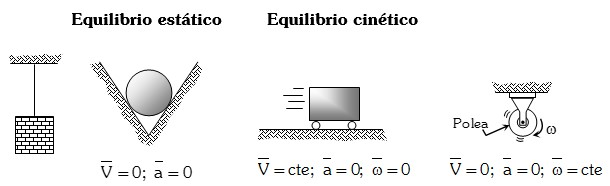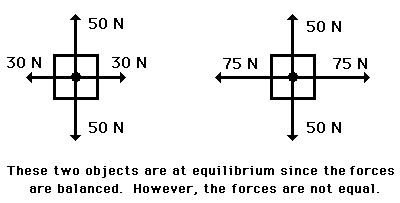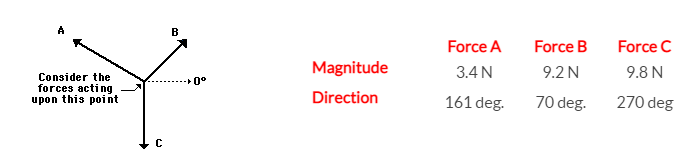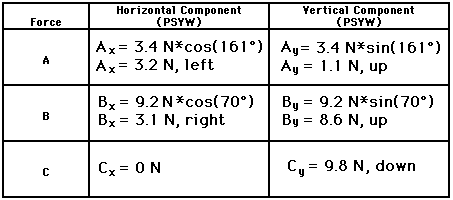# what is statics in physics and how is it present in everyday life?

did you know that static in phys ics is what keeps us safe every time we enter a building or cross a bridge? These are some of the most illustrative examples of statics that we encounter in our day-to-day lives and serve to give you an idea of what statics in physics is.

Engineers use statics to determine, for example, where the supports of a building should go and how many there should be. Or to figure out what type of construction is the best choice for designing a bridge to cross the city river.

Understanding what statics is in physics will even help you to be able to use it the next time you go up a staircase. When you do, you’ll realize that even if an object doesn’t move, there are all kinds of things that affect it, like stress and pressure.

So put on your lab coat and goggles because we’re going to look at what statics means in the branch of physics with applications and exercises that will help you understand it better.

welcome!

## Definition of statics in physics

According to the portal Exercises in Physics, statics is a branch of mechanics, whose objective is the study of the conditions that must be met by aset of forces acting on a body or a rigid system so that it is in mechanical equilibrium.

what does it mean for a body to be in mechanical equilibrium? Basically, two things: that it is in equilibrium when it is at rest (static equilibrium) or that it is in uniform rectilinear motion (kinetic or dynamic equilibrium).

This means that statics in physics refers not only to objects that are completely still, but also to those that move at a constant velocity. Accordingly, the science of statics can tell us a lot about the behavior of things when it comes to balancing, compressing or stretching them. And in the case of buildings and bridges, engineers and architects are more interested in static equilibrium.

Now, according to the formulas of statics, a motionless body does not spontaneously set itself in motion, some external agent is needed to move it. So, we can take as examples of statics in physics:

the attraction of an iron rod if the object is a magnet,
a muscular effort if the object is a mass to be lifted.

In other words, all external agents that disturb the “natural” state of stillness or, as mentioned above, of uniform rectilinear motion, are called forces.Image: ejerciciosdefisica.com

## The force in statics

To understand well what is statics in physics, we must mention the force, since the aim of statics is to establish the total force of the result of all the forces that intervene on a body and that are the ones that achieve the conditions of its equilibrium.

So what is the role of force in statics? Force is the cause capable of modifying the state of stillness or motion of a body; it is also what causes the deformation of the body. The effect produced by the force on a body depends on:

the magnitude,
the direction,
the direction
and the point where it is applied.

Therefore, force is a vector physical quantity. what does this mean? That the force is completely determined by a number, a unit, a direction and a sense, and the number and the unit are known as the modulus. For example, the modulus of wind speed is 3 km/h. And the modulus of a force is a number that indicates its intensity.

Now, what are the symbols for force and statics scientifically? According to the International System of Units, the unit of measurement of the magnitude force is the Newton (N). Forces are symbolized by arrows and the size is directly proportional to the modulus of the force it represents.

## how to calculate statics in physics?

For a system to find the equilibrium point, it must satisfy the three equations of equilibrium:

Sum Fx = 0

Sum Fy = 0

Sum M = 0

In this case, Fx is the force exerted on the horizontal axis and Fy on the vertical axis. And M, the mass, must be zero, since Newton’s first law (F=ma), tells us that if the force and acceleration are 0, so is the sum of the masses.

Today, the methods and calculations of statics in physics have helped in the construction of many engineering works such as buildings, bridges and dams, as well as mechanical devices such as cranes. This is because the first thing architects and engineers must do is to calculate the forces acting on bodies and their components. Statics provides the analytical and graphical procedures needed to describe and identify these unknown forces.

For a body to be considered static, it must be assumed that the body is perfectly rigid and in equilibrium, and the sum of the forces on the object at rest add up to zero. This means that the forces balance each other and do not cause the object to move or rotate.

Consider the following example:Source: Physics Classroom

In the above graph, the two objects are in equilibrium since the forces are balanced even though they do not all have the same strength.

Therefore, the net force is zero and the acceleration is 0 m/s/s/s. For an object to be in equilibrium, it must have an acceleration of zero as mentioned in Newton’s first law. That said, an acceleration of 0 m/s/s/s does not mean that the object is at rest. Rather, it means that a body in equilibrium can be:

At rest
Moving in the same direction and with the same speed

## Statics Exercises

Now that you know what statics is in physics, let’s look at an exercise.

When an object is at rest and in a state of equilibrium, we can say that it is in static equilibrium. A classic laboratory experiment involves hanging an object from two or more strings or ropes and measuring the forces exerted at an angle on the object so that it has a weight.

Let’s look at an example of physical statics:Source: Physics Clasroom

In this case, the object is at the intersection between the three forces and is in equilibrium, since the net force and acceleration are zero.

To calculate the force, the following formulas are used:

F=√ ( F(x)^2+ (F(y)^2)

Where:

F(x) = F cosα

Y

F(y) = F sinα

Applying these formulas to this example:Source: Physics Classroom

The net resultant force is 0 Newtons. This makes sense since the object is in equilibrium. Since we have seen an exercise in physical statics, let’s look at some examples of where it occurs in our day to day lives.

## Examples of statics in physics

### 1. A car on a bridge

An example of statics in physics is a car standing on a bridge, as it exerts a considerable amount of force on the surface between the car and the bridge. However, this force does not cause any change in the state, position or shape of the bridge or the car . Therefore, the sum of the forces is zero.

### 2. Hanging a picture

You don’t need an introduction to carpentry to intuit how statics acts on physics in this example. When we hang an object on the wall, a number of forces act on it. Gravity pulls the body down and, at the same time, the force offered by the wall together with the nail holds the object in position. The painting does not change in state or position as time passes, so it can be considered to be in equilibrium.Source: Unsplash

### 3. A boat floating in the sea

Another example of statics in physics occurs when a boat is on the surface of the water. The gravitational force pulls the boat toward the center of the earth while the buoyant force exerted by the boat pushes it upward. This creates a balance that keeps the body static.

### 4. Pushing on a wall

When an individual applies a pushing force to the surface of a well-constructed wall, the wall does not move. Even if you increase the force you apply, the wall does not change position. This suggests the existence of an opposing force that goes in the opposite direction and does not allow the position or state of the wall to change. This is known as the reaction force and causes the body to be in equilibrium.

### 5. Standing on the floor

Yes. At all times you are a product of static in physics. When a person is standing on a flat surface, the gravitational force and the reaction force exerted by the floor keep them in a static state. These are just a few examples of statics in physics and, as you can see, our world is full of them. can you think of any others?Source: Unsplash

## Brief history of statics

In this section of our article on statics in physics, we are now going to see what was the historical evolution of the principles of statics. The earliest known studies of statics date back more than two thousand years. Although the traces are not many, they are enough to arouse interest and admiration for those who worked for the science of statics in depth.

So we will emphasize two works in particular, which are considered true relics of ancient wisdom: that of Aristotle, who devoted himself to the treatment of mechanical problems, and that of Archimedes, who dealt in his researches with the various problems of equilibrium.

### Aristotle

Yes, we know. You may be wondering what Aristotle has to do with statics because he was neither a physicist nor a mathematician, but a philosopher. Well, his work on this subject was truly admirable for his time, as it tends to establish the principles of statics. According to Aristotle, all motion must encounter resistance.

Therefore, it presupposes the intervention of an action or driving force, which must be greater than its mass. The greater the mass to be moved, the greater the velocity to be applied. However, these principles were not developed with due rigor and he approaches the mechanics of statics in a general way.

### Archimedes

Meanwhile, the first advances in the theory of statics in physics had to be made in other ways. Thus, the beginnings of statics date back to ancient Greece, where more than 2,200 years ago the mathematician Archimedes studied the theory of statics.tic Archimedes studied the application of forces of simple machines, such as a lever or a shaft.

Archimedes was mathematically minded, and set aside general ideas about the motion of bodies and statics, which he considered too complex and inadequate. To make precise and concrete propositions, with a more mathematical sense,

Archimedes preferred to turn his attention to the simplest phenomena and instruments, on which he based all his reasoning and theories about statics. Thus, his treatise contains his studies on the lever and the centers of gravity, aspects that he deals with on the basis of the following hypotheses:

Two equal weights suspended at equal distances from the point of support are in equilibrium.
Two equal weights suspended at different distances are not in equilibrium, and the one suspended at a greater distance begins to descend.
If two weights suspended at certain distances are in equilibrium and one of them ascends slightly, they cease to be in equilibrium and the one that has ascended begins to descend.

This is how the great Greek physicist laid the foundations for the use of leverage and the equilibrium of submerged bodies and gave way to hydrostatics.

### Other important scientists

Later, Leonardo da Vinci and Galileo Galilei would come to leave a legacy of important contributions about statics in physics. In fact, it was the scientist Galileo who determined that no net force was necessary to maintain the motion of a body (dynamic equilibrium).
Likewise, it was Simon Stevin (1548-1620) who noticed the hydrostatic paradox and was the first to describe the equilibrium of bodies on an inclined plane.
Isaac Newton (1642-1727) contributed the three laws of mechanics to the foundations of statics.
D’Alembert left the concept of inertial force. Thanks to his contribution it is possible to study dynamic problems by means of the concept of equilibrium.
Euler and Lagrange developed mathematical techniques that shaped the applications of statics.

We know that the list of engineers and scientists who gave their lives and their contributions to the science of statics have been more than those we have named. how did you like it?

So, you already know what statics is in physics, how it is calculated and some examples present in our lives. Whether you are just learning about it or you are already an experienced connoisseur of the science, it is important for you to learn how this phenomenon builds our world.

Statics in physics is just one part of the vast world of physics. We could write essays full of calculations and examples of this in our lives. It is even enough to look out of your window and see the construction sites and roads of your city to realize how this discipline is present in our routine. Statics in physics is behind all the calculations and civil engineering of our buildings, bridges and more.

If you love physics and science like us, and want to learn more about it, we invite you to visit these YouTube channels to learn much more or these science podcasts that will make you an expert.

good luck!